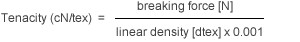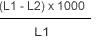# YARN TEST METHODS

Yarn test methods that are used when values such as tenacity, elongation or shrinkage are determined

## 1. TEST ROOM CONDITIONS

The standard according to BISFA is:

• room temperature: 20 ± 2°C
• relative humidity: 65 ± 2%

All tests must be carried out in a conditioned state.

## 2. LINEAR DENSITY

The linear density decitex [dtex] corresponds to the yarn weight in grams of 10'000 meters lengths.

In order to determinde the linear density, a yarn sample of 25 or 100 meters length is wound on a standard reel under a pretension of 0.5 cN/tex and weighed on an analytical scale. Hereafter, the grams per 10'000m yarn are calculated.

## 3. BREAKING FORCE AND ELONGATION AT BREAK

The breaking force leads to the rupture of the thread or yarn. The corresponding value is Newton [N].

The elongation at break is the increase in length produced by stretching a test specimen to the breaking point, expressed as a percentage of the inital length. The test is carried out on a dynmometer with a constant increase in elongation, according to the following conditions:

• length between clamps: 500 mm (or 250 mm)

• test velocity; if elongation at break
• ≤ 8%:     50 mm/min (25 mm/min)
• 8-50%:   500 mm/min (250 mm/min)
• > 50%:   1000 mm/min (500 mm/min)

• pretension: 0.5 cN/tex

## 4. TENACITY (YARNS COUNT TENACITY)

This is calculated from the breaking force as well as linear density and is expressed in centi Newton per tex [cN/tex]:## 5. FORCE AT SPECIFIED ELONGATION (FASE)

This is the force resulting from a defined extension (usually at 2% and 5%).

## 6. HOT AIR SHRINKAGE

This is the shrinkage in length in percent caued by treatment with hot air. We distinguish between free shrinkage and shrinkage under pretension.

### FREE SHRINKAGE

This value defines the change of length after the influence of heat.

Method: After the measurement of length (L1) under 1 cN/tex pretension the samples will be treated without pretension at the defined temperature (between 100°C and 200°C) during 15 minutes. After a cooling time of 1 hour under standard atmospheric conditions, the shrunken length (L2) will be determined again with 1 cN/tex pretension. The residual shrinkage is then calculated according to the following formula:### SHRINKAGE UNDER PRESSION

This value defines the change of length during the influence of heat.

Method: The sample is introduced into the heating channel with a pretension of 0.1 cN/tex at 180°C. The shrinkage will be read in percent directly from a scale.

• Polyamide 6:             15 min at 180°C

• Polyamide 6.6
• < dtex 940:        10 min at 180°C
• ≥ dtex 940:        15 min at 180°C

• Polyester:                    15 min at 180°C

### SHRINKAGE CURVE

The shrinkage curve in our brochure "Product information" was obtained by continuous heating under pretension of 0.1 cN/tex. Due to this, there can be differences to the indicated value in the table.

## 7. HOT AIR SHRINKAGE FORCE

This is the shrinkage force in cN resulting by hot air treatment (180°C).
Pretension: 0.1 cN/tex.

## 8. HOT WATER SHRINKAGE 95°C

This is the shrinkage in length which is caued by hot water. It is calculated as follows:

• On a standard reel, skeins of 1m circumference and approximately 5'000 dtex are prepared and measured at a prention of 1 cN/tex lengthwise = L1

• The skeins are then treated without tension during 15 minutes in hot water (95°C), dried and conditioned at standard conditions.

• The shrunk length is determined again with 1 cN/tex pretension = L2

• The hot water shrinakge in percent is calculated according to the following formula:Looking for a product? We love to serve you well. Find Products
Looking for a product?